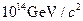Chapter 33, Problem 15PE

Chapter
Section
Textbook Problem

The mass of a theoretical particle that may be associated with the uni?cation of the electroweak and strong forces is(a) How many proton masses is this?(b) How many electron masses is this? (This indicates how extremely relativistic the accelerator would have to be in order to make the particle, and how large the relativistic quantity (would have to be.)

To determine

(a)

Number of proton masses in a theoretical particle associated with unification of

electroweak and strong force.

Explanation

Given:

Mass of theoretical particle =1014 GeV/c2

Formula used:

Number of proton masses = mass of theoretical particle/mass of proton

Calculation:

Mass of theoretical particle =1014 GeV/c2

Mass of proton =938 MeV/c2

Therefore, number of proton masses =1014GeV/c2938MeV/

To determine

(b)

Number of electron masses in a theoretical particle associated with unification of

electroweak and strong force.

Still sussing out bartleby?

Check out a sample textbook solution.

See a sample solution

The Solution to Your Study Problems

Bartleby provides explanations to thousands of textbook problems written by our experts, many with advanced degrees!

Get Started

Find more solutions based on key concepts